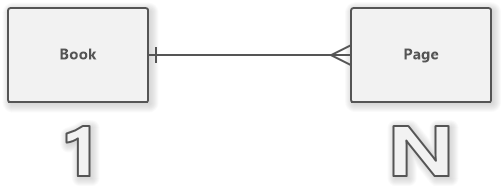# One to Many Relationship (Entity-Relationship Diagram)

A relationship with one-to-many cardinality represents a link between two entities A and B, where one instance of the A entity refers to many instances of the B entity.

A relationship with one-to-many cardinality represents a link between two entities A and B, where one instance of the A entity refers to many instances of the B entity. An instance of the B entity is always associated with exactly one instance of the A entity.

In crow's foot notation, it is depicted as a line with a perpendicular dash on the side of one and a reverted arrow (crow's foot) on the side of many.

## One to Many in Database

A one-to-many relationship between two tables A and B in the database means that a row of table A can be referenced by multiple rows in table B.

A row from table B must reference one row from table A.

## Example of One to Many Relationship

An example of a one-to-many relationship in real life can be a relationship between a book and pages. A book has many pages. A page is associated with exactly one book.

### ER Diagram for One to Many Relationship

The example shows an ER diagram with two entities that are connected with a one-to-many relationship:One to N Relationship

Comment